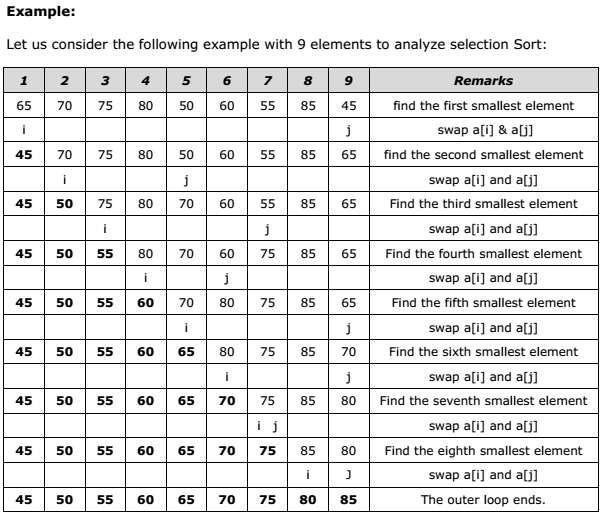Selection sort will not require no more than n-1 interchanges. Suppose x is an array of size n stored in memory. The selection sort algorithm first selects the smallest element in the array x and place it at array position 0; then it selects the next smallest element in the array x and place it at array position 1. It simply continues this procedure until it places the biggest element in the last position of the array.

The array is passed through (n-1) times and the smallest element is placed in its respective position in the array as detailed below:

Pass 1: Find the location j of the smallest element in the array x , x, . . . . x[n-1], and then interchange x[j] with x. Then x is sorted.

Pass 2: Leave the first element and find the location j of the smallest element in the sub-array x, x, . . . . x[n-1], and then interchange x with x[j]. Then x, x are sorted.

Pass 3: Leave the first two elements and find the location j of the smallest element in the sub-array x, x, . . . . x[n-1], and then interchange x with x[j]. Then x, x, x are sorted.

Pass (n-1): Find the location j of the smaller of the elements x[n-2] and x[n-1], and then interchange x[j] and x[n-2]. Then x, x, . . . . x[n-2] are sorted. Of course, during this pass x[n-1] will be the biggest element and so the entire array is sorted.

Time Complexity:

In general we prefer selection sort in case where the insertion sort or the bubble sort requires exclusive swapping. In spite of superiority of the selection sort over bubble sort and the insertion sort (there is significant decrease in run time), its efficiency is also O(n^2) for n data items.You may be interested in:
Data Structures and Algorithms – MCQs.
Data Structures and Algorithms Online Tests